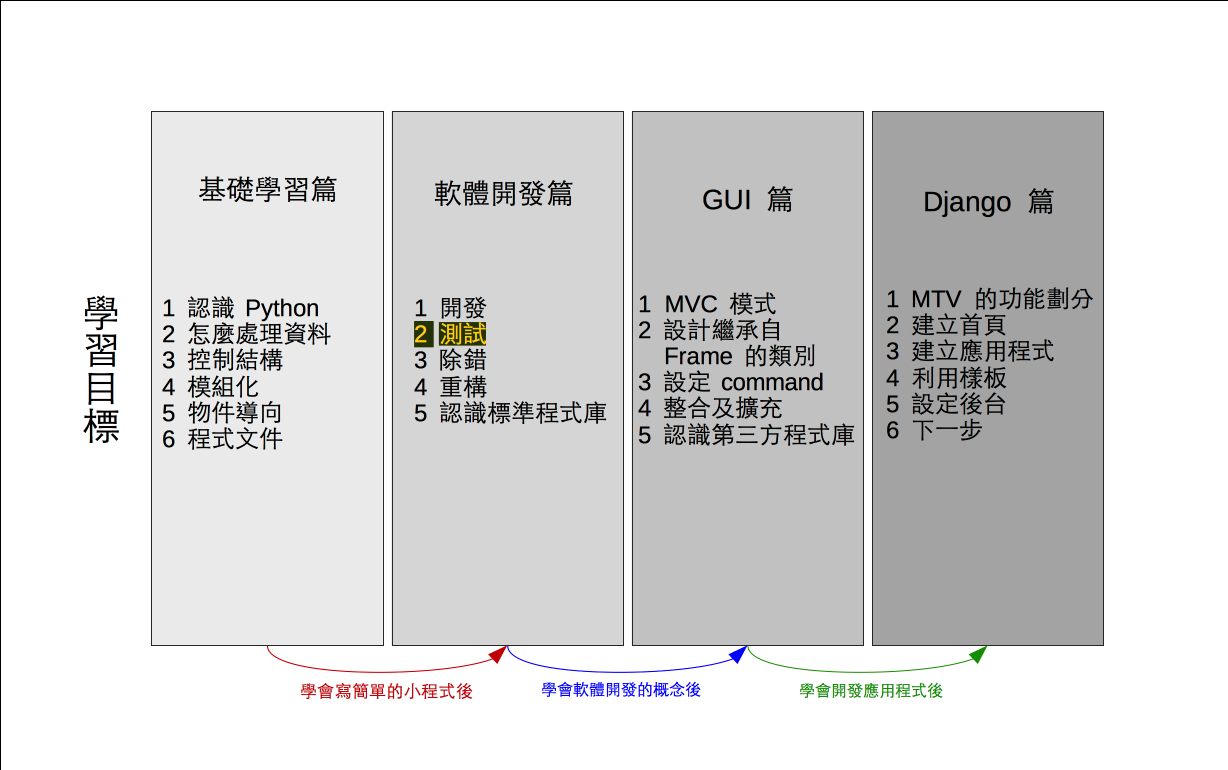# 單元 16 - 繼續測試

～～學習進度表～～Development

Use

Modification

Analysis

Design

Implement

Test

``````# 使用 randint()
import random

# 定義 Encrypt 類別
class Encrypt:
def __init__(self):
self.setcode()

def setcode(self):
# 取得 a 、 b 值
a = random.randint(0, 9)
b = random.randint(0, 9)
# 利用公式建立密碼表
self.code = ""
c = "a"
i = 0
while i < 26:
x = c
y = ord(x) * a + b
m = y % 26
self.code += chr(m + 97)
c = chr(ord(c) + 1)
i += 1

def getcode(self):
return self.code

# 編碼的方法
def toEncode(self, str):
pass

# 解碼的方法
def toDecode(self, str):
pass

# 測試部分
if __name__ == '__main__':
e = Encrypt()
print()
print(e.getcode())
print()

#《程式語言教學誌》的範例程式
# http://kaiching.org/
# 檔名：encrypt03.py
# 功能：示範利用 Python 設計 Encrypt 類別
# 作者：張凱慶 */``````
``````# 使用 randint()
import random``````

``````# 取得 a 、 b 值
a = random.randint(0, 9)
b = random.randint(0, 9)``````

 \$ python encrypt03.py tuvwxyzabcdefghijklmnopqrs \$

 \$ python encrypt03.py nprtvxzbdfhjlnprtvxzbdfhjl \$ python encrypt03.py ffffffffffffffffffffffffff \$ python encrypt03.py \$ python encrypt03.py zabcdefghijklmnopqrstuvwxy \$

1. 軟體的生命週期分成開發、使用、修改三大階段。
2. 開發階段就是對需求分析，然後設計、建置、測試等等。
3. 標準程式庫中 randomrandint() 可產生指定範圍的隨機整數。
4. 設計、建置完軟體的初始版本就進行測試，如果有問題，就重新檢視設計， Encrypt 的數學公式有問題，因此要研究問題出在哪，再回頭修改程式。

1. 軟體的生命週期分成哪些階段？
2. 設計軟體的時候，該不該重視使用者的體驗經驗？
3. 想一想， Encrypt 的數學公式問題可能發生在哪裡？該如何修正呢？

1. 承接上一個單元的猜數字遊戲，將新程式寫在 exercise1601.py 中，把測試答案與猜測陣列的部份寫在函數 test() 裡，結果回傳布林值。
2. 承上題，設計一個函數 ab_counter() 計算 AB 值，用另一個 ab_array 串列紀錄 AB 值，若使用者沒用猜對就印出AB 值，將新程式寫在 exercise1602.py 中。
3. 承上題，加入迴圈直到使用者猜對答案為止，將新程式寫在 exercise1603.py 中。